Probability Distribution Function and Shape

The Bivariate Normal Distribution

A pair of random variables X and Y have a bivariate normal distribution iff their joint probability density is given byfor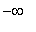< x <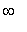and< y <, where> 0,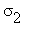> 0, and -1 <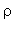< 1.

The following code will draw the density function for the bivariate normal distribution. Note that there are five parameters at work here,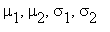and. Try altering these parameters, and see what happens to the distribution. Don't forget to adjust the plot range accordingly, or you might not see anything at all!

> restart:

> with(plots,display,textplot3d):

> mu:=0; mu:=0; sigma:=1; sigma:=1; rho:=0;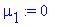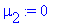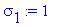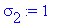> f(x,y):=exp((-1/(2*(1-rho^2)))*(((x-mu)/sigma)^2-2*rho*(x-mu)*(y-mu)/(sigma*sigma)+((y-mu)/sigma)^2))/(2*Pi*sigma*sigma*sqrt(1-rho^2));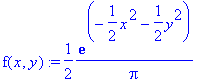> plot3d(f(x,y),x=-3..3,y=-3..3,axes=frame,title="Bivariate Normal");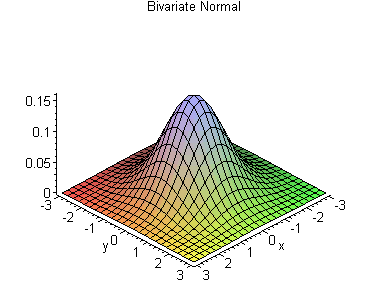Did you notice anything in particular about the joint density after playing with the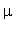's and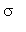's? If you guessed that the's and's are the means and standard deviations for X and Y , you're right! Just to be sure, let's look at the marginal density of X . Unfortunately, Maple doesn't like to use subscripts when assuming conditions for its variables, so we'll have to temporarily endure the use of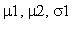and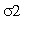for the subscripted versions.

> restart:

> with(student):

> f(x,y):=exp((-1/(2*(1-rho^2)))*(((x-mu1)/sigma1)^2-2*rho*(x-mu1)*(y-mu2)/(sigma1*sigma2)+((y-mu2)/sigma2)^2))/(2*Pi*sigma1*sigma2*sqrt(1-rho^2));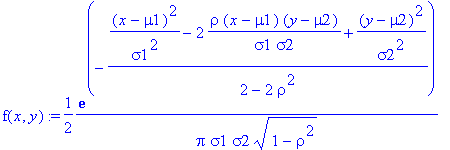> assume(sigma1>0):assume(sigma2>0):

> g(x):=int(f(x,y),y=-infinity..infinity);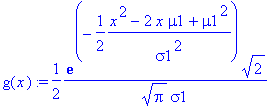But this can be written as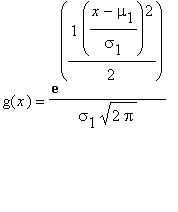, which is simply a normal distribution with meanand standard deviation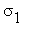. Similarly, the marginal density for Y is a normal distribution with mean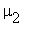and standard deviation.

That leaves us with, whose meaning may have be harder to intuit.is called the correlation coefficient , and it measures how strongly the two random variables vary together. When an expression is found for Cov( X , Y ) and is standardized byand, the expression will simplify to.

> sigma:=value(Doubleint((x-mu1)*(y-mu2)*f(x,y),x=-infinity..infinity,y=-infinity..infinity));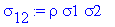> sigma/(sigma1*sigma2);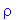Voila!

Now that we have talked about the parameters at some length, let's develop more intuition about how the parameters affect the shape of the density. The next bit of code shows what happens as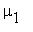changes, ceteris paribus .

> restart:

> with(plots,display,textplot3d):

> mu_start:=-1.5; mu:=0: sigma:=1: sigma:=1: rho:=0: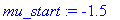> f(x,y):=exp((-1/(2*(1-rho^2)))*(((x-mu1)/sigma)^2-2*rho*(x-mu1)*(y-mu)/(sigma*sigma)+((y-mu)/sigma)^2))/(2*Pi*sigma*sigma*sqrt(1-rho^2)):

> step:=.1;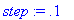> for k from 0 to 30

> do

> mu1:=mu_start+k*step:

> num:=convert(mu1,string):

> mu_value:=textplot3d([-3,2,0.12,`mu is `.num],color=blue);

> density:=plot3d(f(x,y),x=-3..3,y=-3..3);

> pic[k]:=display({density,mu_value})

> od:

> film:=[seq(pic[k],k=0..30)]:

> display(film,insequence=true,axes=frame,title="mu is changing!");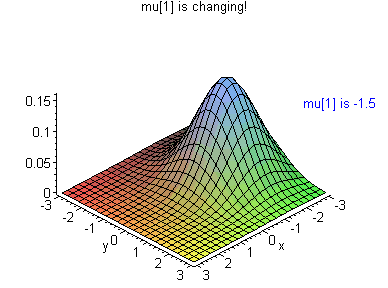Now, let's look at this animation again. This time, however, we will let the standard deviation of Y get smaller asis increasing.

> restart:

> with(plots,display,textplot3d):

> mu_start:=-1.5; mu:=0: sigma:=1: sigma_start:=1; rho:=0: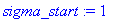> f(x,y):=exp((-1/(2*(1-rho^2)))*(((x-mu1)/sigma)^2-2*rho*(x-mu1)*(y-mu)/(sigma*sigma2)+((y-mu)/sigma2)^2))/(2*Pi*sigma*sigma2*sqrt(1-rho^2)):

> step1:=.1; step2:=.021;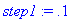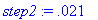> for k from 0 to 30

> do

> mu1:=mu_start+k*step1:

> sigma2:=sigma_start-k*step2:

> num1:=convert(mu1,string):

> num2:=convert(sigma2,string):

> mu_value:=textplot3d([-3,2,0.12,`mu is `.num1],color=blue);

> sigma_value:=textplot3d([2,-3,0.12,`sig is `.num2],color=blue);

> density:=plot3d(f(x,y),x=-3..3,y=-3..3);

> pic[k]:=display({density,mu_value,sigma_value})

> od:

> film:=[seq(pic[k],k=0..30)]:

> display(film,insequence=true,axes=frame,title="sigma is changing, too!");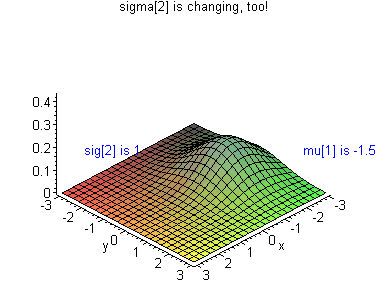The normal curves become tighter and tighter on the Y cross-section! If you can't see this effect clearly, you might want to change your POV by rotating the animation.

Now let's change gears and examine the effect of. We will watch an animation of a bivariate normal distribution wherevaries across the interval (-1, 1).

> restart:

> with(plots,display,textplot3d):

> mu:=0: mu:=0: sigma:=1: sigma:=1: rho_start:=-0.9;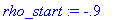> step:=.06;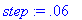> f(x,y):=exp((-1/(2*(1-rho^2)))*(((x-mu)/sigma)^2-2*rho*(x-mu)*(y-mu)/(sigma*sigma)+((y-mu)/sigma)^2))/(2*Pi*sigma*sigma*sqrt(1-rho^2)):

> for k from 0 to 30

> do

> rho:=rho_start+k*step:

> num:=convert(rho,string):

> rho_value:=textplot3d([-3,2,0.34,`rho is `.num],color=blue);

> density:=plot3d(f(x,y),x=-3..3,y=-3..3);

> pic[k]:=display({density,rho_value})

>

> od:

> film:=[seq(pic[k],k=0..30)]:

> display(film,insequence=true,axes=frame,title="rho across (-1,1)");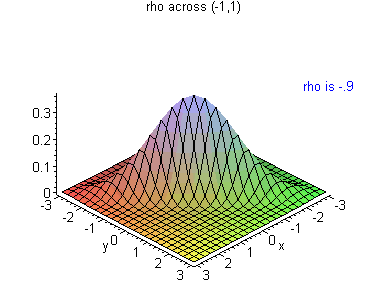The surface starts twisting around in this instance. - a slightly more overhead perspective might help here. At first, X varies negatively with Y , but asincreases, X varies positively with Y .